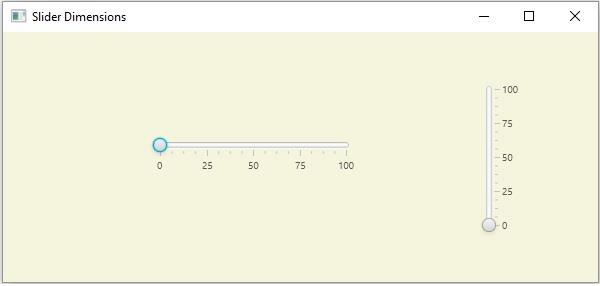# How to change the dimensions of a slider in JavaFX?

JavaFX provides a class known as Slider, this represents a slider component that displays a continuous range of values. This contains a track on which the numerical values are displayed. Along the track, there is a thumb pointing to the numbers. You can provide the maximum, minimum, and initial values of the slider.

In JavaFx you can create a slider by instantiating the javafx.scene.control.Slider class. This class provides three methods to change the dimensions of a slider −

• The setPrefHeight() method − This method accepts a double vale and sets it, as the height of the slider.

• The setPrefWidth() method − This method accepts a double vale and sets it, as the width of the slider.

• The setPrefSize() method − This method accepts two double values as parameters, representing the required width and height of the slider. If you invoke this method the dimensions of the slider will be set to the specified values.

## Example

import javafx.application.Application;
import javafx.geometry.Insets;
import javafx.geometry.Orientation;
import javafx.scene.Group;
import javafx.scene.Scene;
import javafx.scene.control.Slider;
import javafx.scene.layout.HBox;
import javafx.scene.paint.Color;
import javafx.stage.Stage;
public class SliderDimensions extends Application {
public void start(Stage stage) {
//Creating slider1
Slider slider1 = new Slider(0, 100, 0);
slider1.setShowTickLabels(true);
slider1.setShowTickMarks(true);
slider1.setMajorTickUnit(25);
slider1.setBlockIncrement(10);
//Setting the dimensions of the slider
slider1.setPrefWidth(200);
slider1.setPrefHeight(150);
//Creating slider2
Slider slider2 = new Slider(0, 100, 0);
//Setting its orientation
slider2.setOrientation(Orientation.VERTICAL);
slider2.setShowTickLabels(true);
slider2.setShowTickMarks(true);
slider2.setMajorTickUnit(25);
slider2.setBlockIncrement(10);
//Setting the dimensions of the slider
slider2.setPrefSize(200, 150);
//Creating the pane
HBox pane = new HBox(50);
//Preparing the scene
Scene scene = new Scene(new Group(pane), 595, 250, Color.BEIGE);
stage.setTitle("Slider Dimensions");
stage.setScene(scene);
stage.show();
}
public static void main(String args[]){
launch(args);
}
}

## Output